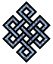#fail2ban bad ip database: ip 106.38.156.204

### | ip database | live view | stats | report | help | api key:

 ip: 106.38.156.204 hostname: 106.38.156.204 country:[CN] China first reported: 16.11.2018 03:51.22 GMT+0200 last reported: 25.02.2019 03:20.15 GMT+0200 time period: 100d 23h 28m 53s total reports: 13 reported by: 2 host(s) filter(s): ssh (7) ssh (6) tor exit node no badips.com db Lookup## port scan of '106.38.156.204':

[-hide]
```# Nmap 6.40 scan initiated Fri Nov 16 03:52:01 2018 as: /usr/bin/nmap -sU -sS -O 106.38.156.204
Nmap scan report for 106.38.156.204
Host is up (0.18s latency).
Not shown: 1987 filtered ports
PORT     STATE         SERVICE
22/tcp   open          ssh
8001/tcp closed        vcom-tunnel
135/udp  open|filtered msrpc
136/udp  open|filtered profile
137/udp  open|filtered netbios-ns
138/udp  open|filtered netbios-dgm
139/udp  open|filtered netbios-ssn
445/udp  open|filtered microsoft-ds
1026/udp open|filtered win-rpc
1027/udp open|filtered unknown
1433/udp open|filtered ms-sql-s
1434/udp open|filtered ms-sql-m
No exact OS matches for host (If you know what OS is running on it, see http://nmap.org/submit/ ).
TCP/IP fingerprint:
OS:SCAN(V=6.40%E=4%D=11/16%OT=22%CT=8001%CU=%PV=N%G=Y%TM=5BEE3534%P=x86_64-
OS:pc-linux-gnu)SEQ(SP=FF%GCD=1%ISR=10A%TI=Z%CI=I%TS=8)OPS(O1=M5B4ST11NW7%O
OS:2=M5B4ST11NW7%O3=M5B4NNT11NW7%O4=M5B4ST11NW7%O5=M5B4ST11NW7%O6=M5B4ST11)
OS:WIN(W1=7120%W2=7120%W3=7120%W4=7120%W5=7120%W6=7120)ECN(R=Y%DF=Y%TG=40%W
OS:=7210%O=M5B4NNSNW7%CC=Y%Q=)T1(R=Y%DF=Y%TG=40%S=O%A=S+%F=AS%RD=0%Q=)T2(R=
OS:N)T3(R=N)T4(R=Y%DF=Y%TG=40%W=0%S=A%A=Z%F=R%O=%RD=0%Q=)T5(R=Y%DF=Y%TG=40%
OS:W=0%S=Z%A=S+%F=AR%O=%RD=0%Q=)T6(R=Y%DF=Y%TG=40%W=0%S=A%A=Z%F=R%O=%RD=0%Q
OS:=)T7(R=N)U1(R=N)IE(R=Y%DFI=N%TG=40%CD=S)

OS detection performed. Please report any incorrect results at http://nmap.org/submit/ .
# Nmap done at Fri Nov 16 04:10:44 2018 -- 1 IP address (1 host up) scanned in 1123.32 seconds
```
```Σ = 72 | Δt = 0.01587700843811s
```